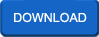## Electrical Machines Notes

 Forming Electrical NetworksSelf-assembly of millimeter-scale polyhedra, with surfaces patterned with solder,dots, wires, and light-emitting diodes, generated electrically functional,three-dimensional networks. The patterns of dots and wires controlled the,structure of the networks formed; both parallel and serial connections were,generated.
Rate this Book:
 Synchronous MachinesWhat does one look like?, How does it work?, What is the model?, How does one find the parameters? How are Synchronous machines used?, What's missing?
Rate this Book:
 Induction motorsWhat does one look like?, How does it work?, What is the model?, How does one find the,parameters?, How are induction machines used?, What's missing?
Rate this Book:
 TransformersWhat does one look like?, How does it work?, What is the model?, How does one find the parameters?, How are transformers used? What's missing?
Rate this Book:
 Magnetic CircuitsAnalogue of electric circuit., Relationship to inductance, Terminology Magnetic materials
Rate this Book:
 Phasor DiagramsRepresentation of a sinusoidal quantity, Magnitude and Phase, Can rotate, but relative phases remain the same. Constant frequency, CCW positive.
Rate this Book: